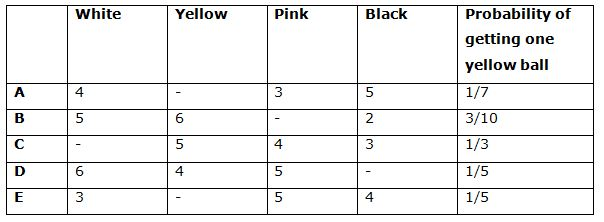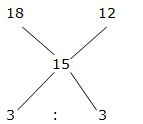# IBPS PO Mains 2018 – Quantitative Aptitude Questions Day-9

Dear Readers, Bank Exam Race for the Year 2018 is already started, To enrich your preparation here we have providing new series of Practice Questions on Quantitative Aptitude – Section. Candidates those who are preparing for IBPS PO Mains 2018 Exams can practice these questions daily and make your preparation effective.

[WpProQuiz 4051]

Directions (Q. 1 – 5): Each question contains Quantity I and Quantity II. Read the contents clearly and answer your questions accordingly.

1)

Quantity I: The length of the rectangle is thrice the side of a square whose area is 324 Sq. m. The breadth of the rectangle is 10 m less than the length of the rectangle. Find the area of rectangle?

Quantity II: The perimeter of a rectangle is 212 m. The difference between the length and breadth of the rectangle is 18 m. Find the area of a rectangle?

a) Quantity I > Quantity II

b) Quantity I ≥ Quantity II

c) Quantity II > Quantity I

d) Quantity II ≥ Quantity I

e) Quantity I = Quantity II or Relation cannot be established

2)

Quantity I: The ratio between the downstream and upstream speed of the boat is 4 : 3. The boat travels 64 km downstream in 8 hours. Find the speed of the boat in still water

Quantity II: The boat travels 72 km downstream in 8 hours. The speed of the stream is 3 km/hr. Find the speed of the boat in still water?

a) Quantity I > Quantity II

b) Quantity I ≥ Quantity II

c) Quantity II > Quantity I

d) Quantity II ≥ Quantity I

e) Quantity I = Quantity II or Relation cannot be established

3)

Quantity I: Two trains are moving in opposite directions at 40 km/hr and 60 km/hr. Train lengths are 250 m and 200 m respectively. The time taken by the slower train to cross the faster train is?

Quantity II: The length of Mumbai express is 170 m and that of Sathapthi express is 180 m. These two trains are running in the same direction with the velocities of 60 km/hr and 80 km/hr respectively. The time taken by them to cross each other is?

a) Quantity I > Quantity II

b) Quantity I ≥ Quantity II

c) Quantity II > Quantity I

d) Quantity II ≥ Quantity I

e) Quantity I = Quantity II or Relation cannot be established

4)

Quantity I: The present age of Anitha is 15 years more the present age of Rahul. After 10 years the ratio of ages of Rahul and Anitha will be 7 : 10, then find the age of Anitha, after 4 years?

Quantity II: The present age of Naveen is thrice the present age of Priyanka. The ratio between the present ages of Gourav and Priyanka is 5 : 3. If the age of Gourav after 6 years will be 21 years, then find the sum of the present age of Naveen, Priyanka and Gourav?

a) Quantity I > Quantity II

b) Quantity I ≥ Quantity II

c) Quantity II > Quantity I

d) Quantity II ≥ Quantity I

e) Quantity I = Quantity II or Relation cannot be established

5) A person invested a sum of Rs. 35600 in two schemes A and B which offers interest at the rate of 18% and 12 % respectively. If the total interest received from these schemes after one year is Rs. 5340 then,

Quantity I: Sum of money invested in scheme A?

Quantity II: Sum of money invested in scheme B?

a) Quantity I > Quantity II

b) Quantity I ≥ Quantity II

c) Quantity II > Quantity I

d) Quantity II ≥ Quantity I

e) Quantity I = Quantity II or Relation cannot be established

Directions (Q. 6 – 10) Study the following information carefully and answer the given questions.

The following table shows the number of 4 different color balls in 6 different bags.6) If 3 balls are taken out randomly from bag A, then find the probability of getting at least one pink ball from bag A?

a) 247/425

b) 78/217

c) 199/364

d) 123/289

e) None of these

7) If 4 balls are taken out randomly from bag C, then find the probability of getting different color balls from bag C?

a) 12/91

b) 45/77

c) 33/125

d) 127/245

e) None of these

8) If 4 balls are taken out randomly from bag D, then find the probability of getting 2 pink balls from bag D?

a) 56/121

b) 123/285

c) 92/157

d) 70/323

e) None of these

9) Find the ratio between the total number of balls in bag B to that of bag E?

a) 5: 6

b) 4: 3

c) 7: 8

d) 5: 4

e) None of these

10) Total number of pink balls in all the bags together is what percentage of total number of yellow balls in all the bags together?

a) 120 %

b) 80 %

c) 150 %

d) 100 %

e) 90 %

Directions (1-5):

Quantity I:

Area of the square = a2 = 324

Side of the square (a) = 18m

Length of the rectangle = 54m

Area of the rectangle = 54*44= 2376 Sq. m

Quantity II:

The perimeter of a rectangle = 212 m

2(l + b) = 212

l + b = 106 —> (1)

l – b = 18 —> (2)

By solving the equation (1) and (2), we get,

Length = 62 m, Breadth = 44 m

Area of the rectangle = 62*44 = 2728 Sq. m

Quantity I < Quantity II

Quantity I:

Downstream speed= 64/8 = 8 km/hr

Upstream speed= 8*(3/4) = 6 km/hr

Speed of boat in still water = ½ *(8+6) = 7 km/hr

Quantity II:

Downstream speed= 72/8= 9 km/hr

Downstream speed = Speed of boat in still water + speed of stream

Let the speed of boat be x

=> x + 3 = 9

=> x= 6 km/hr

Speed of boat in still water = 6 km/hr

Quantity I > Quantity II

Quantity I:

T= D/S

T = (250 + 200)/[(40 + 60)*5/18]

T = 450/(100*(5/18)) = 16.2 sec

Quantity II:

T = D/S

T = (170 + 180)/[(80 – 60)*(5/18)]

T = 350/(20*(5/18))

T = 63 sec

Quantity I < Quantity II

Quantity I:

Present age of Anitha = 15 + Present age of Rahul

After 10 years, The ratio of ages of Rahul and Anitha = 7 : 10(7x, 10x)

10x – 10 = 15 + 7x – 10

10x – 10 = 7x + 5

3x = 15

X = 5

The age of Anitha, after 4 years = 10x – 6 = 50 – 6 = 44 years

Quantity II:

The ratio of present age of Naveen and Priyanka = 3 : 1

The ratio of present age of Gourav and Priyanka = 5 : 3

The present age of Naveen, Priyanka and Gourav = 9 : 3 : 5

The age of Gourav, after 6 years = 21 years

The present age of Gourav = 15 years

5’s = 15

1’s = 3

Required sum = 17*3 = 51 years

Quantity I < Quantity II

Percentage of interest received from these schemes

= 5340*100/35600

= 15%

By the rule of allegation,= > 1 : 1

2’s = 35600

1’s = 17800

Quantity I:

Sum of money invested in scheme A= Rs. 17800

Quantity II:

Sum of money invested in scheme B = Rs. 17800

Quantity I = Quantity II

Directions (6-10):

Let the yellow ball be X,

Probability of getting one yellow ball = 1/7

XC1/(12 + X)C1 = 1/7

X/(12 + X) = 1/7

7X = 12 + X

6X = 12

X = 2

Yellow balls in bag A = 2 balls

Total probability = 14C3

The probability of getting at least one pink ball

= > 1 – P(none is pink ball)

Probability of getting none is pink ball

= > 11C3 /14C3 = 165/364

The probability of getting at least one pink ball

= > 1 – (165/364) = 199/364

Let the white ball be X,

Probability of getting one yellow ball = 1/3

5C1/(12 + X)C1 = 1/3

5/(12 + X) = 1/3

15 = 12 + X

X = 3

The total white balls in bag C = 3

Total probability = 15C4

Required probability = 3C1 and 5C1 and 4C1 and 3C1

The probability of getting different color balls

= > [3C1 and 5C1 and 4C1 and 3C1]/15C4

= > 12/91

Let the black ball be X,

Probability of getting one yellow ball = 1/5

4C1/(15 + X)C1 = 1/5

4/(15 + X) = 1/5

20 = 15 + X

X = 5

Total number of black balls = 5

Total probability = 20C4

Required probability = 5C2 and 15C

The probability of getting 2 pink balls from bag D

= > (5C2 and 15C)/20C4

= > 70/323

Let the pink ball in bag B be X,

Probability of getting one yellow ball = 3/10

6C1/(13 + X)C1 = 3/10

6/(13 + X) = 3/10

13 + X = 20

X = 7

The total number of pink ball in bag B = 7 balls

Total balls in bag B = 5 + 6 + 7 + 2 = 20

Let the yellow ball in bag E be Y,

Probability of getting one yellow ball = 1/5

YC1/(12 + Y)C1 = 1/5

Y/(12 + Y) = 1/5

5Y = 12 + Y

4Y = 12

Y = 3

The total number of yellow ball in bag E = 3 balls

Total balls in bag E = 3 + 3 + 5 + 4 = 15

Required ratio = 20: 15 = 4: 3

Let the pink ball in bag B be X,

Probability of getting one yellow ball = 3/10

6C1/(13 + X)C1 = 3/10

6/(13 + X) = 3/10

13 + X = 20

X = 7

The total number of pink ball in bag B = 7 balls

Total number of pink balls in all the bags together

= > 3 + 7 + 4 + 5 + 5 = 24

Let the yellow ball in bag A be Y,

Probability of getting one yellow ball = 1/7

YC1/(12 + Y)C1 = 1/7

Y/(12 + Y) = 1/7

7Y = 12 + Y

6Y = 12

Y = 2

Yellow balls in bag A = 2 balls

Let the yellow ball in bag E be Z,

Probability of getting one yellow ball = 1/5

ZC1/(12 +Z)C1 = 1/5

Z/(12 + Z) = 1/5

5Z = 12 + Z

4Z = 12

Z = 3

The total number of yellow ball in bag E = 3 balls

Total number of yellow balls in all the bags together

= > 2 + 6 + 5 + 4 + 3 = 20

Required % = (24/20)*100 = 120 %

Daily Practice Test Schedule | Good Luck

 Topic Daily Publishing Time Daily News Papers & Editorials 8.00 AM Current Affairs Quiz 9.00 AM Current Affairs Quiz (Hindi) 9.30 AM IBPS PO Mains – Reasoning 10.00 AM IBPS PO Mains– Quantitative Aptitude 11.00 PM Vocabulary (Based on The Hindu) 12.00 PM IBPS PO Mains – English Language 1.00 PM IBPS PO/Clerk – GK 3.00 PM Daily Current Affairs Updates 5.00 PM IBPS Clerk Prelims – Reasoning 6.00 PM IBPS Clerk Prelims – Reasoning (Hindi) 6.30 PM IBPS Clerk Prelims – Quantitative Aptitude 7.00 PM IBPS Clerk Prelims – Quantitative Aptitude (Hindi) 7.30 PM IBPS Clerk Prelims – English Language 8.00 PM